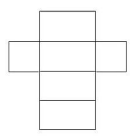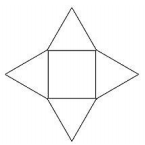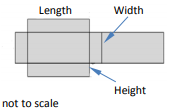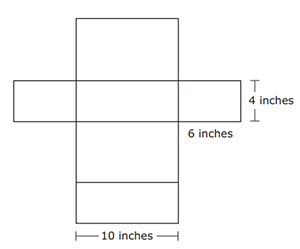# MAFS.6.G.1.4Archived StandardExport Print
Represent three-dimensional figures using nets made up of rectangles and triangles, and use the nets to find the surface area of these figures. Apply these techniques in the context of solving real-world and mathematical problems.
General Information
Subject Area: Mathematics
Domain-Subdomain: Geometry
Cluster: Level 2: Basic Application of Skills & Concepts
Cluster: Solve real-world and mathematical problems involving area, surface area, and volume. (Supporting Cluster) -

Clusters should not be sorted from Major to Supporting and then taught in that order. To do so would strip the coherence of the mathematical ideas and miss the opportunity to enhance the major work of the grade with the supporting clusters.

Date of Last Rating: 02/14
Status: State Board Approved - Archived
Assessed: Yes
Test Item Specifications

• Assessment Limits :
Numbers in items must be positive rational numbers. Three-dimensional figures are limited to rectangular prisms, triangular prisms, rectangular pyramids, and triangular pyramids.
• Calculator :

No

• Context :
Allowable
Sample Test Items (4)
• Test Item #: Sample Item 1
• Question:

A net is shown.What three-dimensional figure is represented by the net?

• Difficulty: N/A
• Type: MC: Multiple Choice

• Test Item #: Sample Item 2
• Question:

A net is shown.What three-dimensional figure is represented by the net?

• Difficulty: N/A
• Type: MC: Multiple Choice

• Test Item #: Sample Item 3
• Question: The surface area of a rectangular prism is 115 square inches. The net of the prism is shown.What are the possible dimensions of the prism?

• Difficulty: N/A
• Type: MC: Multiple Choice

• Test Item #: Sample Item 4
• Question:

Carl is shipping a cardboard box that is a rectangular prism. The net of Carl's box is shown.What is the area of cardboard, in square inches, required for Carl's box?

• Difficulty: N/A
• Type: EE: Equation Editor

## Related Courses

This benchmark is part of these courses.
1205010: M/J Grade 6 Mathematics (Specifically in versions: 2014 - 2015, 2015 - 2022, 2022 and beyond (current))
1205020: M/J Accelerated Mathematics Grade 6 (Specifically in versions: 2014 - 2015, 2015 - 2020, 2020 - 2022, 2022 and beyond (current))
1204000: M/J Foundational Skills in Mathematics 6-8 (Specifically in versions: 2014 - 2015, 2015 - 2022, 2022 and beyond (current))
0101010: M/J Two-Dimensional Studio Art 1 (Specifically in versions: 2014 - 2015, 2015 - 2022, 2022 and beyond (current))
0101020: M/J Two-Dimensional Studio Art 2 (Specifically in versions: 2014 - 2015, 2015 - 2022, 2022 and beyond (current))
0101040: M/J Three-Dimensional Studio Art 1 (Specifically in versions: 2014 - 2015, 2015 - 2022, 2022 and beyond (current))
0101050: M/J Three-Dimensional Studio Art 2 (Specifically in versions: 2014 - 2015, 2015 - 2022, 2022 and beyond (current))
7812015: Access M/J Grade 6 Mathematics (Specifically in versions: 2014 - 2015, 2015 - 2018, 2018 - 2022, 2022 and beyond (current))
0101025: M/J Two-Dimensional Studio Art 2 & Career Planning (Specifically in versions: 2014 - 2015, 2015 - 2019, 2019 - 2022, 2022 and beyond (current))
0101005: M/J Exploring Two-Dimensional Art (Specifically in versions: 2014 - 2015, 2015 - 2022, 2022 and beyond (current))
0101026: M/J Two-Dimensional Studio Art 3 (Specifically in versions: 2014 - 2015, 2015 - 2022, 2022 and beyond (current))
0101035: M/J Exploring Three-Dimensional Art (Specifically in versions: 2014 - 2015, 2015 - 2022, 2022 and beyond (current))
0101060: M/J Three-Dimensional Studio Art 3 (Specifically in versions: 2014 - 2015, 2015 - 2022, 2022 and beyond (current))

## Related Access Points

Alternate version of this benchmark for students with significant cognitive disabilities.

## Related Resources

Vetted resources educators can use to teach the concepts and skills in this benchmark.

## Formative Assessments

Windy Pyramid:

Students are asked to use a net to find the surface area of a triangular pyramid.

Type: Formative Assessment

Rust Protection:

Students are asked to use a net to find the surface area of a rectangular prism.

Type: Formative Assessment

Pyramid Project:

Students are asked to draw a net of a three-dimensional figure.

Type: Formative Assessment

Skateboard Ramp:

Students are asked to draw a net of a three-dimensional figure.

Type: Formative Assessment

## Lesson Plans

Wrapping Up Geometry (Lesson 2 of 3):

Lesson 2 of 3 on Surface Area, this lesson is designed to take students from the previous lesson of recognizing nets of rectangular prisms and finding areas of its individual faces, to recognizing nets of triangular prisms and finding areas of its individual faces in order to find the surface area of the prism.

Type: Lesson Plan

Wrapping Up Geometry (Lesson 1 of 3):

This lesson is the first of three in a unit on surface area. This lesson provides a foundation for understanding the concept of surface area by introducing nets of rectangular prisms.

Type: Lesson Plan

Netty People and Pets:

Students will learn what a "net" is, draw nets of three dimensional shapes, accurately calculate the surface area of their nets, and put them together to create an original person or pet.

Type: Lesson Plan

Hands-On! Rectangular Prisms:

Students create surface area nets with graph paper and work with manipulative cubes to decide if there is a relationship between surface area and volume in rectangular prisms.

Type: Lesson Plan

What's on the Surface?:

In this activity, students will work in groups to evaluate the measurements of shapes that form three-dimensional composite shapes to compute the surface area.

Type: Lesson Plan

Students will be able to match a 3-D shape with its net, then using the net, they will find the surface area of the shape. They will then be able to apply this knowledge to solve real world application problems, finishing up with a design contest.

Type: Lesson Plan

Building a Tree House:

This MEA will have students determining the safest and most cost effective material to use when building a tree house.They will do this by calculating surface area and determining cost.

Type: Lesson Plan

Surface Area of Prisms and Pyramids:

In this lesson students will find the surface area of three-dimensional figures. Students will use nets made up of rectangles and triangles to calculate the surface area of rectangular prisms, triangular prisms, and square pyramids.

Type: Lesson Plan

How Much Paint Will It Take?:

This is a guided inquiry lesson to help students gain greater understanding of the relationship between 2-dimensional and 3-dimensional shapes. Students create right rectangular and triangular prisms and problem-solve how to find the flat 2-dimensional surface area. Students are asked to figure out how many party favors (prisms) can be painted with a quart of glow-in-the-dark paint.

Type: Lesson Plan

Box It Up, Wrap It Up (Surface Area of Rectangular Prisms):

In this introductory lesson to surface area, students will make connections between area of two dimensional figures and calculating the surface area of rectangular prisms using nets, within the context of wrapping birthday presents! Math is Fun :)

Type: Lesson Plan

Wrapping Up Geometry (Lesson 3 of 3):

This lesson is 3 of 3 in a unit and is primarily formative in nature but I have attached a summative assessment with this lesson for students to take during the following class period.

During the lesson, students will be reviewing for their assessment on the surface area formula for a rectangular prism.

Type: Lesson Plan

Lola's Landscaping MEA:

In this Model Eliciting Activity, MEA, students are asked to develop a procedure to fit the most amount of rectangular prism plant packages on one sheet of cardboard, using nets and surface area.

Type: Lesson Plan

Formula Detective: Finding the Surface Area of a 3D Figure:

This lesson allows students to derive the formulas for 3D figures by having them build models for nets.

Type: Lesson Plan

Analyzing Polyhedra:

Students will construct several simple polyhedra, then count the number of faces, edges, and vertices. These data should suggest Euler's formula.

Type: Lesson Plan

Three-Dimensional Play Dough:

This hands-on lesson is a review for three-dimensional figures. The students make models of three-dimensional figures and then use these play dough models to count the vertices, edges, and faces.

Type: Lesson Plan

Boxing Candles:

This lesson is designed for 7th grade students and is best suited for advanced students. It can be used (with modifications) in the general education classroom for 7th grade or in an advanced 6th grade classroom. In this MEA, students select jars for candles based on a variety of factors and then design boxes to contain the jars.

Type: Lesson Plan

Surface Area and Volume:

In this activity, students adjust the dimensions of either a rectangular or triangular prism and the surface area and volume are calculated for those dimensions. Students can also switch into compute mode where they are given a prism with certain dimensions and they must compute the surface area and volume. The application keeps score so students can track their progress. This application allows students to explore the surface area and volume of rectangular and triangular prisms and how changing dimensions affect these measurements. This activity also includes supplemental materials, including background information about the topics covered, a description of how to use the application, and exploration questions for use with the java applet.

## Student Center Activity

Students can practice answering mathematics questions on a variety of topics. With an account, students can save their work and send it to their teacher when complete.

Type: Student Center Activity

## Teaching Idea

Cylinder Surface Discovery:

"Students create their own cylinder and find the surface area by combining the areas of the two circles and the rectangular piece" (from Beacon Lesson Plan Library).

Type: Teaching Idea

## Tutorials

Nets of 3-Dimensional Figures:

This video demonstrates how to construct nets for 3-D shapes.

Type: Tutorial

Finding Surface Area of a Rectangular Prism :

This video demonstrates using a net to find surface area.

Type: Tutorial

## Unit/Lesson Sequences

Using Surface Area and Volume to Design Candy Cartons:

In these two 50-minute lessons, students will work in pairs or small groups to design cartons that can contain specifically sized candies. Upon completion of these lessons, students will have selected appropriate mathematical models for an unstructured problem, identified constraints and variables, and worked with two and three dimensional shapes to solve a problem involving capacity and surface area.

Type: Unit/Lesson Sequence

Three Dimensional Shapes:

In this interactive, self-guided unit on 3-dimensional shape, students (and teachers) explore 3-dimensional shapes, determine surface area and volume, derive Euler's formula, and investigate Platonic solids. Interactive quizzes and animations are included throughout, including a 15 question quiz for student completion.

Type: Unit/Lesson Sequence

## STEM Lessons - Model Eliciting Activity

Boxing Candles:

This lesson is designed for 7th grade students and is best suited for advanced students. It can be used (with modifications) in the general education classroom for 7th grade or in an advanced 6th grade classroom. In this MEA, students select jars for candles based on a variety of factors and then design boxes to contain the jars.

Building a Tree House:

This MEA will have students determining the safest and most cost effective material to use when building a tree house.They will do this by calculating surface area and determining cost.

Lola's Landscaping MEA:

In this Model Eliciting Activity, MEA, students are asked to develop a procedure to fit the most amount of rectangular prism plant packages on one sheet of cardboard, using nets and surface area.

## MFAS Formative Assessments

Pyramid Project:

Students are asked to draw a net of a three-dimensional figure.

Rust Protection:

Students are asked to use a net to find the surface area of a rectangular prism.

Skateboard Ramp:

Students are asked to draw a net of a three-dimensional figure.

Windy Pyramid:

Students are asked to use a net to find the surface area of a triangular pyramid.

## Student Resources

Vetted resources students can use to learn the concepts and skills in this benchmark.

## Student Center Activity

Students can practice answering mathematics questions on a variety of topics. With an account, students can save their work and send it to their teacher when complete.

Type: Student Center Activity

## Tutorials

Nets of 3-Dimensional Figures:

This video demonstrates how to construct nets for 3-D shapes.

Type: Tutorial

Finding Surface Area of a Rectangular Prism :

This video demonstrates using a net to find surface area.

Type: Tutorial

## Parent Resources

Vetted resources caregivers can use to help students learn the concepts and skills in this benchmark.